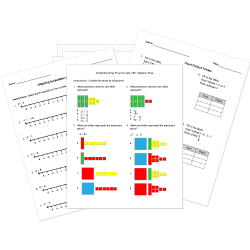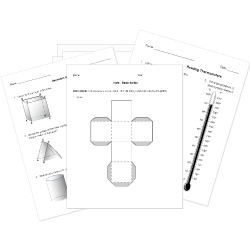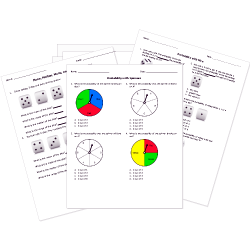Tweet# Printable High School Math Tests and Worksheets

Assess student understanding of algebra, geometry, statistics, and trigonometry concepts with these math worksheets for high school students.

High school math worksheets labeled with are accessible to Help Teaching Pro subscribers only. Become a Subscriber to access hundreds of standards aligned worksheets.

Don't see a high school math worksheet you need? Use our Test Maker™ to create your own printable that fits your needs. Browse math questions or use our advanced search to find existing questions while filtering by grade levels and keywords. Customize your test further by creating your own assessment questions.

Related: Online Self-Paced Math Lessons

## High School Algebra WorksheetsUse these high school algebra worksheets which cover Pre-Algebra, Algebra I, and Algebra II to gauge student comprehension of algebra topics including: linear and quadratic equations, inequalities, functions, exponents, radicals, and logarithms.

## High School Geometry WorksheetsInvestigate lines, angles, circles, triangles, polygons, perimeter, volume, and the coordinate plane, among other topics with these geometry worksheets.

## High School Statistics WorksheetsThe ability to estimate, interpret, and manipulate data is an essential skill. Strengthen student understanding of probability and statistics concepts with these worksheets.

## High School Trigonometry Worksheets

Explore topics such as trigonometric ratios and functions, Sine and Cosine Law, trigonometric identities, the unit circle, and radians and degrees with these worksheets.

## High School Calculus Worksheets

These worksheets give students a chance to practice calculus topics, including limits, differentiation, tangent lines, and integration.